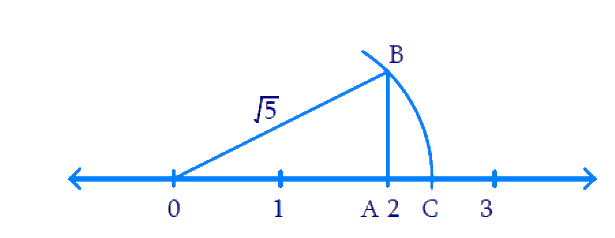# Ex.1.2 Q3 Number System Solution - NCERT Maths Class 9

Go back to  'Ex.1.2'

## Question

Show how \begin{align}\sqrt 5 \end{align} represented on the number line.

Video Solution
Number Systems
Ex 1.2 | Question 3

## Text Solution

What is known?

Integer $$5$$.

What is unknown?

Point representing \begin{align}\sqrt 5 \end{align} on the number line.

Steps:

We shall write $$5$$ on the sum of two squares in the form \begin{align}5=2^{2}+1^{2}=4+1=5 \end{align}. This shows we need to construct a right triangle with sides $$2$$ and $$1$$ units. So the hypotenuse becomes \begin{align} \sqrt{5} \end{align} units on the number line. We shall proceed as follows.

DiagramOn the number line take $$2$$ units from $$O$$ and represent the point as $$A$$. At $$A$$ draw the perpendicular and mark $$B$$ such that \begin{align} AB =1\end{align} unit with $$O$$ as center and \begin{align} OB\end{align} as radius. Draw an arc to cut the number line at $$C$$. Now $$C$$ represents \begin{align}\sqrt 5 \end{align}.

In $$\Delta OAB,$$

\begin{align} O{{B}^{2}}&=O{{A}^{2}}+A{{B}^{2}} \\ &={{2}^{2}}+{{1}^{2}} \\ &=5 \\ \therefore OB &=\sqrt{5}=OC \\ \end{align}

Video Solution
Number Systems
Ex 1.2 | Question 3

Learn from the best math teachers and top your exams

• Live one on one classroom and doubt clearing
• Practice worksheets in and after class for conceptual clarity
• Personalized curriculum to keep up with school